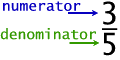Home Math Calculators Math  Flashcards Math Games Math  Homework Math Lessons Math Terms Math Worksheets Math Tools
You Are In Math Glossary of Terms

# Fraction

A fraction is a part of a whole, like a half, a third, a quarter, etc.

Example :Half of an apple is a fraction of an apple.

The top number in a fraction is called the numerator;

the bottom number in a fraction is called the denominator.